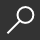## Lie Algebras : Theory and Algorithms, Volume 56 by W. a. de Graaf

Overview -
The aim of the present work is two-fold. Firstly it aims at a giving an account of many existing algorithms for calculating with finite-dimensional Lie algebras. Secondly, the book provides an introduction into the theory of finite-dimensional Lie algebras. These two subject areas are intimately related. First of all, the algorithmic perspective often invites a different approach to the theoretical material than the one taken in various other monographs (e.g., 42], 48], 77], 86]). Indeed, on various occasions the knowledge of certain algorithms allows us to obtain a straightforward proof of theoretical results (we mention the proof of the Poincar -Birkhoff-Witt theorem and the proof of Iwasawa's theorem as examples). Also proofs that contain algorithmic constructions are explicitly formulated as algorithms (an example is the isomorphism theorem for semisimple Lie algebras that constructs an isomorphism in case it exists). Secondly, the algorithms can be used to arrive at a better understanding of the theory. Performing the algorithms in concrete examples, calculating with the concepts involved, really brings the theory of life.

local_shippingFor DeliveryIn Stock.
This item is Non-Returnable.
FREE Shipping for Club Members helpNew & Used Marketplace 5 copies from \$77.00

## More About Lie Algebras by W. a. de Graaf

### Overview

The aim of the present work is two-fold. Firstly it aims at a giving an account of many existing algorithms for calculating with finite-dimensional Lie algebras. Secondly, the book provides an introduction into the theory of finite-dimensional Lie algebras. These two subject areas are intimately related. First of all, the algorithmic perspective often invites a different approach to the theoretical material than the one taken in various other monographs (e.g., 42], 48], 77], 86]). Indeed, on various occasions the knowledge of certain algorithms allows us to obtain a straightforward proof of theoretical results (we mention the proof of the Poincar -Birkhoff-Witt theorem and the proof of Iwasawa's theorem as examples). Also proofs that contain algorithmic constructions are explicitly formulated as algorithms (an example is the isomorphism theorem for semisimple Lie algebras that constructs an isomorphism in case it exists). Secondly, the algorithms can be used to arrive at a better understanding of the theory. Performing the algorithms in concrete examples, calculating with the concepts involved, really brings the theory of life.

This item is Non-Returnable.

### Details

• ISBN-13: 9780444501165
• ISBN-10: 0444501169
• Publisher: North-Holland
• Publish Date: February 2000
• Page Count: 408
• Dimensions: 9.21 x 6.14 x 0.94 inches
• Shipping Weight: 1.64 pounds

Series: North-Holland Mathematical Library #56Related Categories